Home > GC > Chapter 8 > Lesson 8.2.1 > Problem8-65

8-65.
1.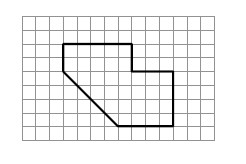the shape at right. 8-65 HW eTool (Desmos). Homework Help ✎

1. Find the area and perimeter of the shape.

2. On graph paper, enlarge the figure so that the linear scale factor is 3. Find the area and perimeter of the new shape.

3. What is the ratio of the perimeters of both shapes? What is the ratio of the areas?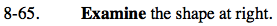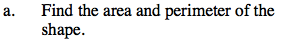Use the graph to find the lengths.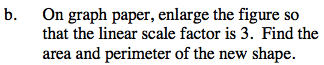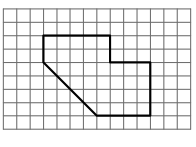Perimeter ≈ 25.7 units

Mutiply each of the dimensions of this figure by 3 to draw the new, larger figure.

Perimeter ≈ 77 units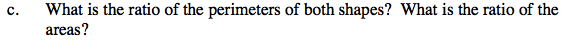Remember that the ratio of the perimeters is not the same as the ratio of the areas.

Use the eTool below for help with this problem.
Click the link at right for the full version of the eTool: GC 8-65 HW eTool